Physic Tutorials

# Notes on Direct Current for Cambridge A level and UTME

Notes on Direct Current for Cambridge A level and UTME

Direct current : flow of charges in the circuit is in the same direction all the time from higher potential to lower potential.

Appropriate circuit symbol

1. switch2. Cell, voltmeter, ammeter, resistor, variable resistor etc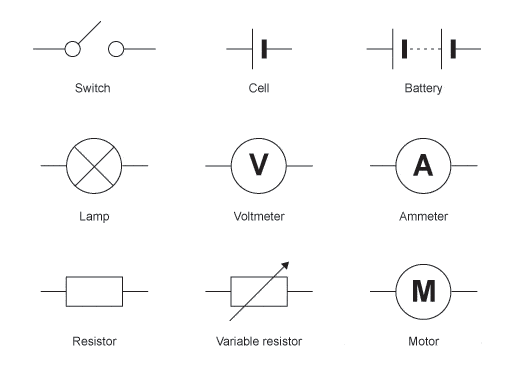Circuit diagram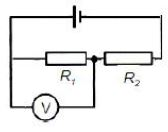Kirchhoff’s First Law: The sum of the currents entering a junction is always equal to the sum of the currents leaving it(conservation of electric charge)

Kirchhoff’s Second Law: The sum of the electromotive forces in a closed circuit is equal to the sum of the potential differences (conservation of energy)

Derive, using Kirchhoff’s laws, a formula for the combined resistance of two or more resistors in series

Series arrangement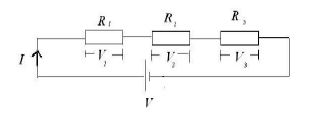Resistors are said to be connected in series if current can flow from one resistor to another without branching.

V = V1 + V2 + V3

V1 = IR1

V2 = IR2

V3 = IR3

V = IR

IRt = IR1 + IR2 + IR3

Note

in series arrangement same current flows through the resistors but different voltage

Rt = R1 + R2 + R3

Parallel arrangement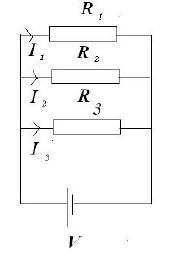When resistors are connect in parallel current branches out but the voltage are the same for all the resistors

I = I1 + I2 + I3

I1 = V / R1

I2 = V / R2

I3 = V / R3

I = V / Rt

V / Rt = V/R1 + V/R2 + V/ R3

1 / Rt = 1 / R1 + 1 / R2 + 1 / R3

Example

Two cells of e.m.f. E1 and E2 and negligible internal resistance are connected into the network in the figure belowThe currents in the network are as indicated in Fig. above
Use Kirchhoff’s laws to state the relation
(i) between currents I1, I2 and I3,

(ii) between E2, R, I2 and I3 in loop BCXYB,

(iii) between E1, E2, R, I1 and I2 in loop ABCXYZA.

Solution

According to Kirchhoff’s First Law: The sum of the currents entering a junction is always equal to the sum of the currents leaving it

Let junction B be our reference point

I1 is entering

I3 is leaving

I2 is entering

I3 = I1 + I2

(ii)

loop BCXYB

following anti-clockwise loop

conventionally current moves from positive terminal and “enters” negative terminal

But in E2 current leaves the negative terminal i.e E2 will be negative

I2 and I3 will also be negative because it opposes the direction of the loop

-E2 = -I2R – I3R

E2 = I2R +I3R

(iii)

loop ABCXYZA

following the anti-clockwise loop

Current leaves negative terminal of E2( this will give us -E2) and enters the negative terminal of E1( this will give us +E1)

I1 will be positive because it is in the direction of the loop

Resistors on A and Z are in series so current I1 flows without branching

I2 will be negative because it opposes the direction of the loop

E1 – E2 = I1R + I1R – I2R

E1 – E2 = 2I1R – I2R

Show an understanding of the use of a potential divider circuit as a source of variable p.d.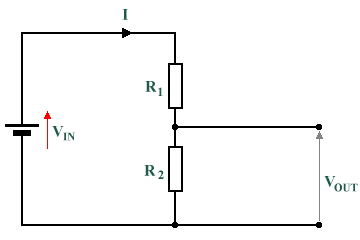Vin = V1 + V2

V1 = IR1

V2 = IR2

Vin =IR1 + IR2

Vin = I(R1 +R2)

I = Vin / (R1 + R2)

V2 = Vin / (R1 + R2) * R2

V2 = Vout

Vout = R2 / (R1 + R2) * VoutUse of thermistors and light-dependent resistors in potential dividers to provide a potential difference that is dependent on temperature and illumination respectivelyThermistor as temperature sensorTemperature against the resistance of a thermistor### Bolarinwa Olajire

A lecturer, Educationist, PhD student at FUNAAB, and a Blogger.